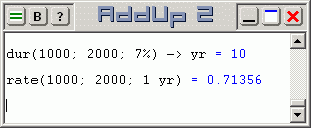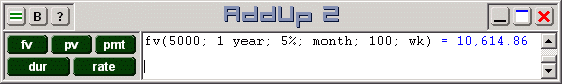# Financial Calculations with AddUp 2

Financial operations are performed with AddUp by entering the financial annuity function to evaluate. A short expression can be typed in and edited at will, then the Enter key causes it to be evaluated. It is not necessary to use buttons for this, what needs to be evaluated can be directly entered in the work area. There are five financial annuity functions:

• fv: Future Value
• pv: Present Value
• pmt: Payment
• rate: Annual Interest Rate
• dur: Duration

The most suitable output format for operations that return an amount (fv, pv and pmt) is naturally currency. A better format for annual rates and durations is the common automatic format. Select the desired option from the Output Format menu.

Financial functions can be used to calculate annuities that cover any conceivable time period so it is necessary to provide a time unit (otherwise values default to seconds). In the simpler cases, annuity functions require three or four parameters and they are calculated using monthly compounding periods.

Using function fv, we can calculate the future value of a thousand dollars invested for 1 year (either "year" or "yr" can be used as a time unit) at five percent yields 1,051.16 dollars. A time unit must be given, but it can be "month" or "mo" instead or a year, or even "wk" or "dy" if this is what is needed.

Function pv gives the present value. We can calculate the present value of a thousand dollars to be 932.58 if it will be received one year from now given a seven percent annual interest rate.

 The pmt (payment) function determines the required regular payment that must be made in order to turn a specified initial value into another after a specified amount of time under a given interest rate. Payments are assumed to be made monthly unless specified otherwise (see below). Here we see that given a five percent interest rate, we can accumulate a thousand dollars starting from zero after a year if we make payments of 81.44 each month.The duration of an annuity investment that will turn one amount into another under a specified interest rate is obtained with the dur function. Since time is expressed in seconds, the result is then converted in the desired time unit (usually either day, week, month or year) by adding the conversion operator and the desired unit. We can see that it takes ten years to double a thousand dollars given 7% interest rate.

The rate function gives the interest rate required to turn one amount into another in the specified amount of time. Doubling a thousand dollars within a year would require a 71% interest rate (0.71356). Good luck finding this!

The above financial calculations assume the most common case of monthly periods for both payments and compounding interest. But there are many more possibilities. Interest could be compounded semi-annually. Payments could be made weekly or bi-weekly and either at the begining or at the end of this payment period. Durations could be expressed in months instead of years, and so on. The same five financial annuity functions can handle all these special cases just by using more parameters. When longer expressions are needed, you can select the Financial Annuity panel from the menu in order to use buttons. The advantage of using buttons is that they fill in a set of default values for you in the work area and you can then simply edit the content. This saves a good deal of typing and it provides a reminder of what each parameter stands for.Clicking the fv button loads the work area with its namesake function. Default parameters are also written except for the initial (present) value. The default duration is one year, annual interest rate is 5%, compounding period in monthly. Two more parameters refer to the regular annuity payment amount and period; these values are set to zero for the amount and the payment period is a month. Using these default values on a thousand dollars gives a formula of fv(1000; 1 year; 5%; month; 0; month). This is equivalent to just fv(1000; 1 year; 5%) as previously used and a 1,051.16 result is obtained as before.

Changing the amount and the default values is simple. Using 5000 as initial value, a duration of 18 months and a payment of 100 each month, we have fv(5000; 18 mo; 5%; month; 100; month) which yields 7,253.77. The same 5000 present value for a year, supplemented with weekly deposits of 100 is expressed with fv(5000; 1 year; 5%; month; 100; wk): we have 10,614.86 by the end of the year.

The other financial calculator buttons provide similar functionality. Default parameters that are relevant to each function are filled in. One final parameter that is rarely used in omitted: a final argument value of 1 can be tagged in to indicate that payments take place at the begining of a payment period instead of at the end (the default case). Refer to the AddUp documentation for details on all financial functions and their parameters.